Variational and Topological Methods: Theory, Applications, Numerical Simulations, and Open Problems.
Electron. J. Diff. Eqns., Conference 21 (2014), pp. 101-127.

### Differentiability properties of p-trigonometric functions Petr Girg, Lukas Kotrla

Abstract:
p-trigonometric functions are generalizations of the trigonometric functions. They appear in context of nonlinear differential equations and also in analytical geometry of the p-circle in the plain. The most important p-trigonometric function is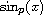. For p>1, this function is defined as the unique solution of the initial-value problemfor any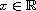. We prove that the-th derivative ofcan be expressed in the form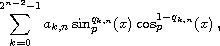on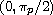, where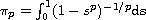, and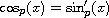. Using this formula, we proved the order of differentiability of the function. The most surprising (least expected) result is that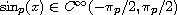if p is an even integer. This result was essentially used in the proof of theorem, which says that the Maclaurin series ofconverges on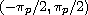if p is an even integer. This completes previous results that were known e.g. by Lindqvist and Peetre where this convergence was conjectured.

Published February 10, 2014.
Math Subject Classifications: 34L10, 33E30, 33F05.
Key Words: p-Laplacian; p-trigonometry; analytic functions; approximation.

Show me the PDF(446 K), TEX and other files for this article.Petr Girg Department of Mathematics, University of West Bohemia Univerzitni 22, 30614 Plzen, Czech Republic email: pgirg@kma.zcu.cz Lukas Kotrla Department of Mathematics, University of West Bohemia Univerzitni 22, 30614 Plzen, Czech Republic email: kotrla@students.zcu.cz